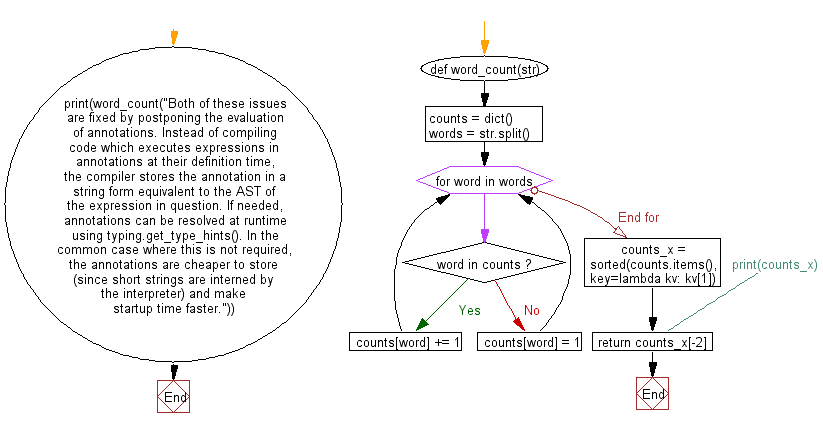﻿ Python: Find the second most repeated word in a given string - w3resource# Python: Find the second most repeated word in a given string

## Python String: Exercise-56 with Solution

Write a Python program to find the second most repeated word in a given string.

Sample Solution:-

Python Code:

``````def word_count(str):
counts = dict()
words = str.split()

for word in words:
if word in counts:
counts[word] += 1
else:
counts[word] = 1

counts_x = sorted(counts.items(), key=lambda kv: kv)
#print(counts_x)
return counts_x[-2]

print(word_count("Both of these issues are fixed by postponing the evaluation of annotations. Instead of compiling code which executes expressions in annotations at their definition time, the compiler stores the annotation in a string form equivalent to the AST of the expression in question. If needed, annotations can be resolved at runtime using typing.get_type_hints(). In the common case where this is not required, the annotations are cheaper to store (since short strings are interned by the interpreter) and make startup time faster."))
```
```

Sample Output:

```('of', 4)
```

Pictorial Presentation:Flowchart:## Visualize Python code execution:

The following tool visualize what the computer is doing step-by-step as it executes the said program:

Python Code Editor:

Have another way to solve this solution? Contribute your code (and comments) through Disqus.

What is the difficulty level of this exercise?

Test your Programming skills with w3resource's quiz.

﻿

## Python: Tips of the Day

Check if a given key already exists in a dictionary:

In is the intended way to test for the existence of a key in a dict.

```d = {"key1": 10, "key2": 23}

if "key1" in d:
print("this will execute")

if "nonexistent key" in d:
print("this will not")
```

If you wanted a default, you can always use dict.get():

```d = dict()

for i in range(100):
key = i % 10
d[key] = d.get(key, 0) + 1
```

and if you wanted to always ensure a default value for any key you can either use dict.setdefault() repeatedly or defaultdict from the collections module, like so:

```from collections import defaultdict

d = defaultdict(int)

for i in range(100):
d[i % 10] += 1
```

but in general, the in keyword is the best way to do it.

Ref: https://bit.ly/2XPMRyz